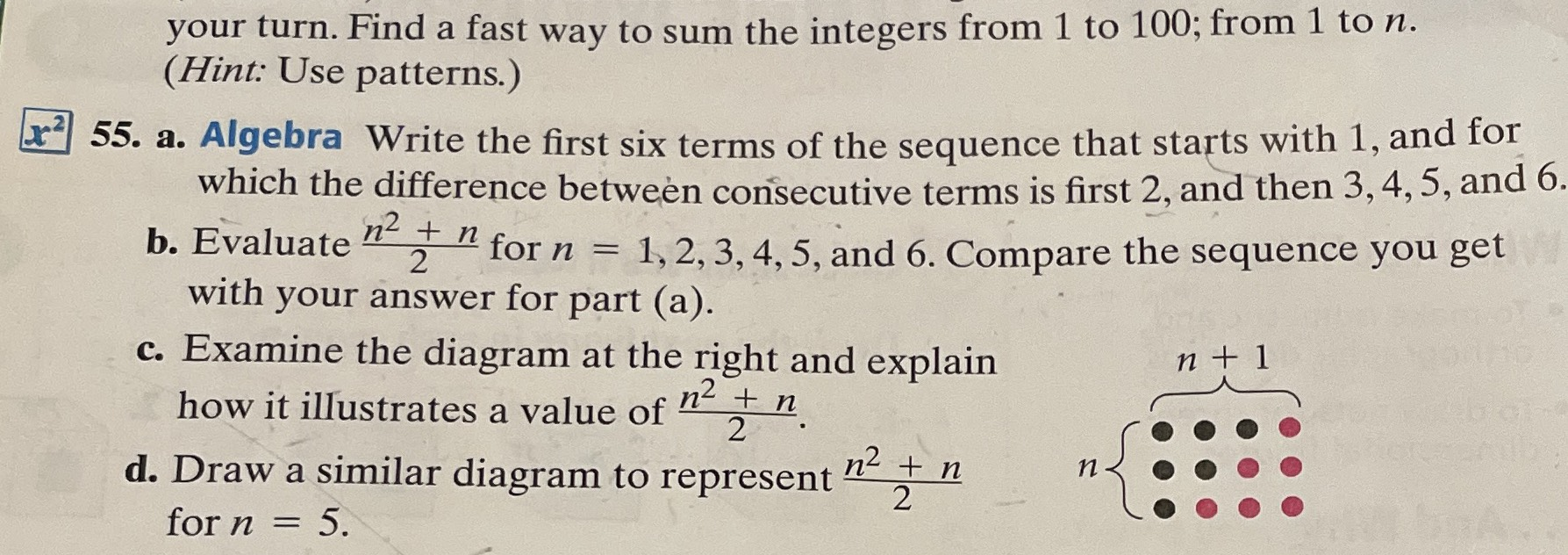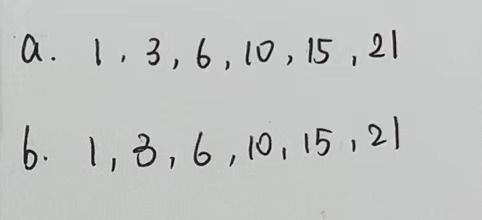### ¿Todavía tienes preguntas de matemáticas?

Pregunte a nuestros tutores expertos
Algebra
Pregunta55. a. Algebra Write the first six terms of the sequence that starts with $$1$$ , and for which the difference between consecutive terms is first $$2$$ , and then $$3,4,5$$ , and $$6$$ .

b. Evaluate $$\frac { n ^ { 2 } + n } { 2 }$$ for $$n = 1,2,3,4,5$$ , and 6. Compare the sequence you get with your answer for part (a).

c. Examine the diagram at the right and explain how it illustrates a value of $$\frac { n ^ { 2 } + n } { 2 }$$ .

d. Draw a similar diagram to represent $$\frac { n ^ { 2 } + n } { 2 }$$ for $$n = 5$$ .